NEED HELP? AQA GCSE digital textbooks From the makers of Grade Gorilla GGebooks.com(Opens in a new tab)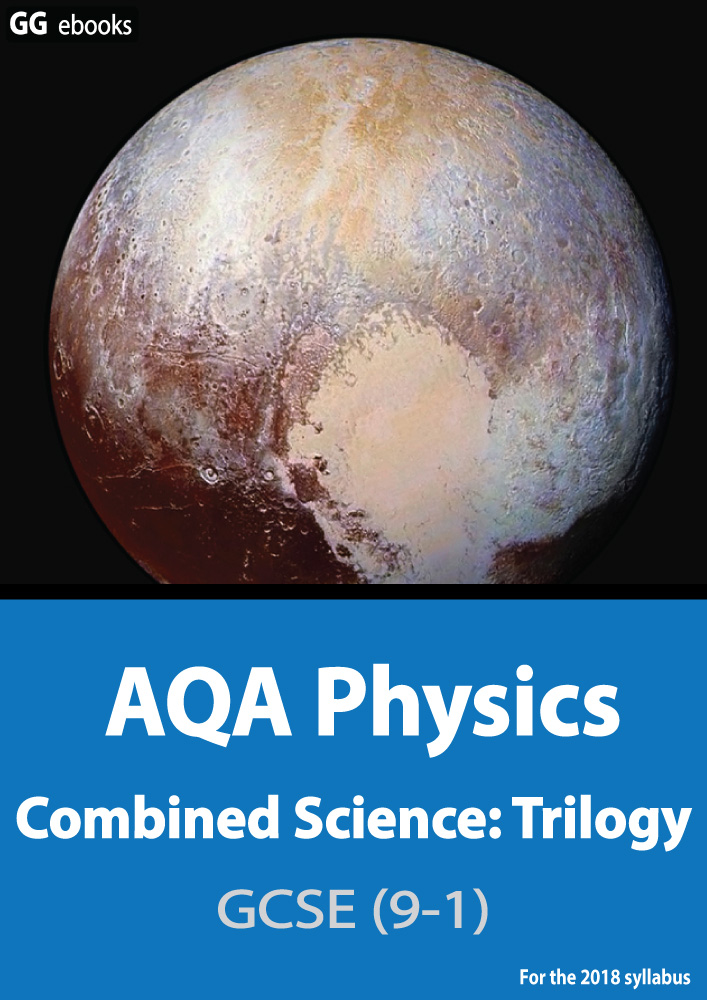Which component matches these symbols?
1.2.3.4. Which of these 4 circuits can be used to measure how the current varies with a change in voltage across a bulb?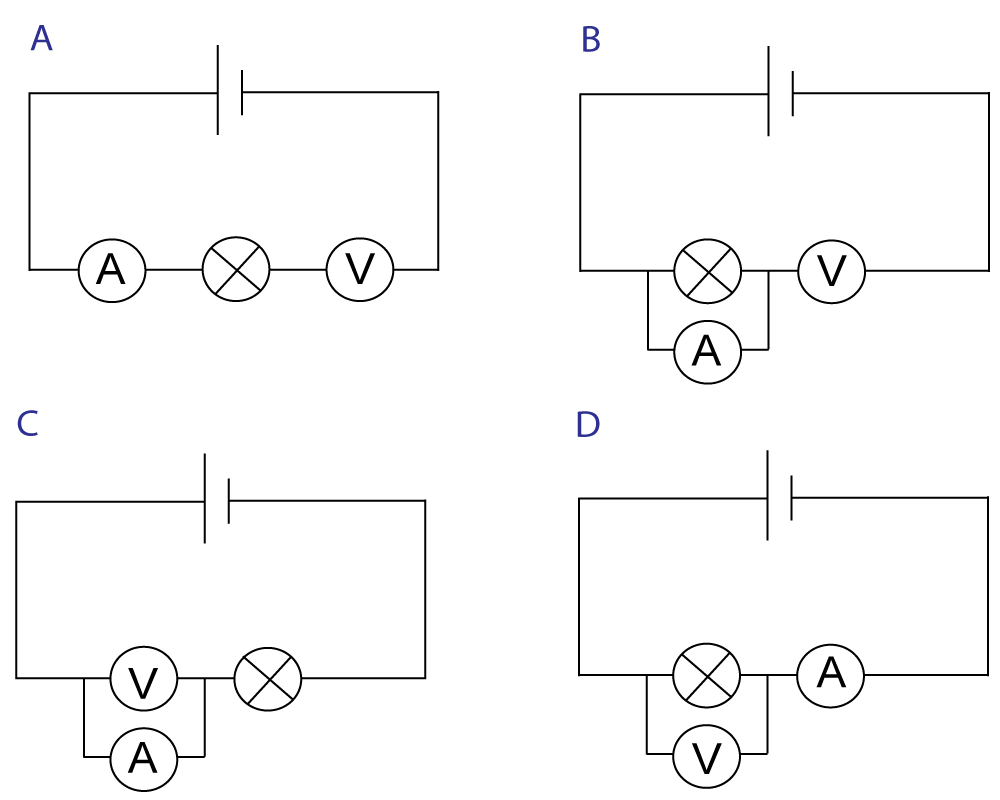Which components have the following characteristic graphs - showing how the current varies as the voltage is changed?
5.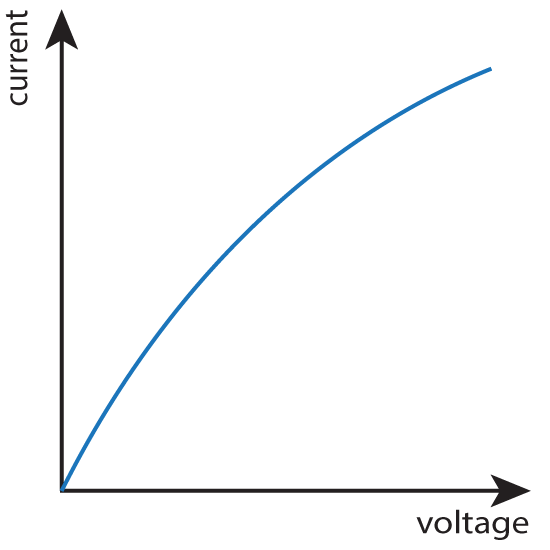6.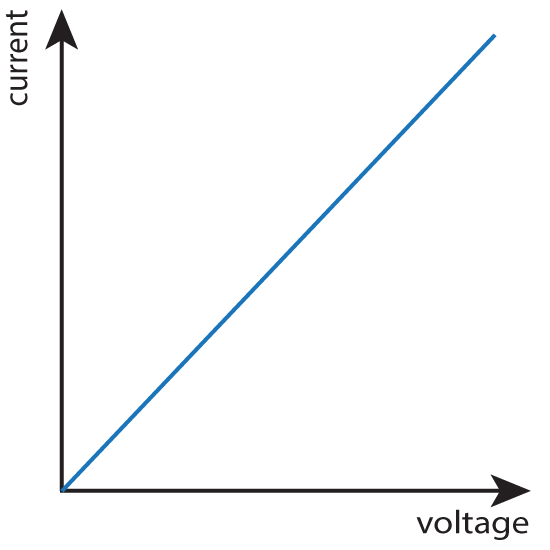7.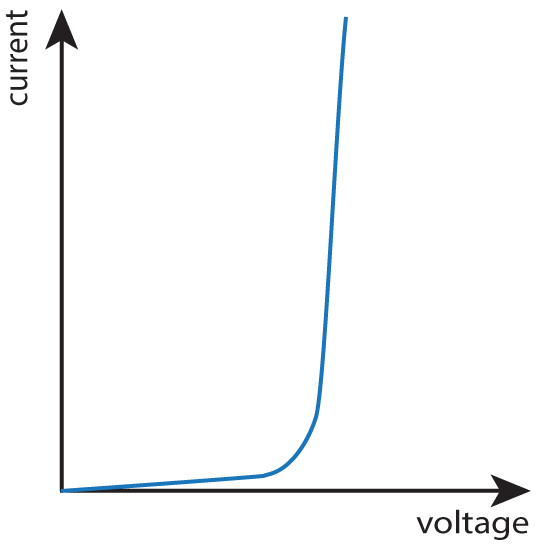8.Which of these answers gives the correct way of connecting ammeters and voltmeters?

 Ammeter Voltmeter A) Series Series B) Parallel Parallel C) Series Parallel D) Parallel Series

A student is experimenting with identical bulbs and 6V cells.

The bulb in this circuit below has a current of 2A passing through it when connected to a single 6V cell as shown.What are the approximate currents in the following circuits using the same bulbs and cells as shown above?

9.10.11.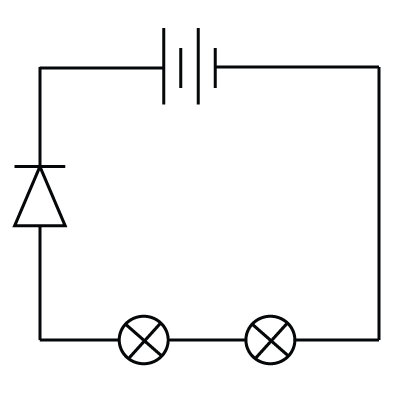12. Two resistors of resistance R are connected in parallel as shown. here.The total resistance will be

• A) less than R
• B) R
• C) greater than R
• D) exactly 2R

The table below shows measurements taken on a selection of resistors. What are the missing values?

 Voltage (V) Current (A) Resistance (Ω) 13. 12 4 ? 0.33 3 4 12 48 14. 60 ? 30 120000 33.33 0.03 2000 15. ? 30 120 300 0.0083 0.5 60